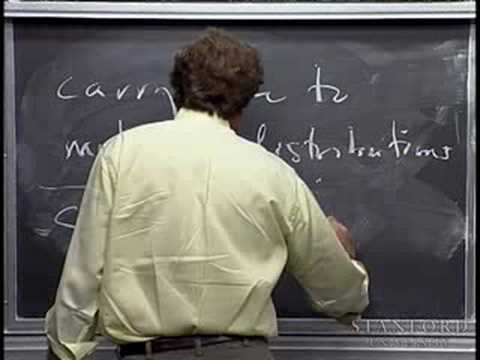Stanford

# The Fourier Transforms and Its Applications

The Fourier transform is a tool for solving physical problems. In this course the emphasis is on relating the theoretical principles to solving practical engineering and science problems. Topics include: The Fourier transform as a tool for solving physical problems. Fourier series, the Fourier transform of continuous and discrete signals and its properties. The Dirac delta, distributions, and generalized transforms. Convolutions and correlations and applications; probability distributions, sampling theory, filters, and analysis of linear systems. The discrete Fourier transform and the FFT algorithm. Multidimensional Fourier transform and use in imaging. Further applications to optics, crystallography. (from see.stanford.edu )

Instructor